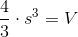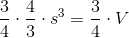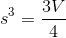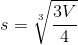# ISEE Upper Level Quantitative : How to find the volume of a pyramid

## Example Questions

### Example Question #1 : Solid Geometry

Pyramid 1 has a square base with sidelength; its height is.

Pyramid 2 has a square base with sidelength; its height is.

Which is the greater quantity?

(a) The volume of Pyramid 1

(b) The volume of Pyramid 2

(b) is greater.

It is impossible to tell from the information given.

(a) is greater.

(a) and (b) are equal.

(b) is greater.

Explanation:

Use the formula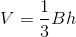on each pyramid.

(a)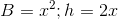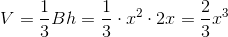(b)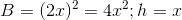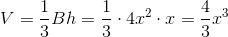Regardless of, (b) is the greater quantity.

### Example Question #2 : Solid Geometry

Which is the greater quantity?

(a) The volume of a pyramid with height 4, the base of which has sidelength 1

(b) The volume of a pyramid with height 1, the base of which has sidelength 2

(a) and (b) are equal.

(a) is greater.

It is impossible to tell from the information given.

(b) is greater.

(a) and (b) are equal.

Explanation:

The volume of a pyramid with heightand a square base with sidelengthis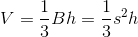.

(a) Substitute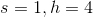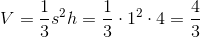(b) Substitute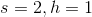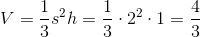The two pyramids have equal volume.

### Example Question #3 : Solid Geometry

Which is the greater quantity?

(a) The volume of a pyramid whose base is a square with sidelength 8 inches

(b) The volume of a pyramid whose base is an equilateral triangle with sidelength one foot

It is impossible to tell from the information given.

(a) and (b) are equal.

(a) is greater.

(b) is greater.

It is impossible to tell from the information given.

Explanation:

The volume of a pyramid is one-third of the product of the height and the area of the base. The areas of the bases can be calculated, but no information is given about the heights of the pyramids. There is not enough information to determine which one has the greater volume.

### Example Question #4 : Solid Geometry

A pyramid with a square base has height equal to the perimeter of its base. Its volume is. In terms of, what is the length of each side of its base?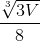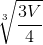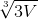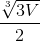Explanation:

The volume of a pyramid is given by the formula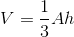whereis the area of its base andis its height.

Letbe the length of one side of the square base. Then the height is equal to the perimeter of that square, so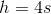and the area of the base is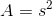So the volume formula becomes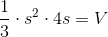Solve for: